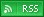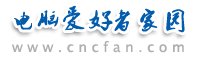电脑爱好者，提供IT资讯信息及各类编程知识文章介绍，欢迎大家来本站学习电脑知识。 最近更新 | 联系我们站内搜索：

# VBScript Sub 过程和Function 过程

Sub 过程
Sub 过程是包含在 Sub 和 End Sub 语句之间的一组 VBScript 语句，执行操作但不返回值。Sub 过程可以使用参数（由调用过程传递的常数、变量或表达式）。如果 Sub 过程无任何参数，则 Sub 语句必须包含空括号 ()。

Sub ConvertTemp()
temp = InputBox("请输入华氏温度。", 1)
MsgBox "温度为 " & Celsius(temp) & " 摄氏度。"
End Sub

Function 过程
Function 过程是包含在 Function 和 End Function 语句之间的一组 VBScript 语句。Function 过程与 Sub 过程类似，但是 Function 过程可以返回值。Function 过程可以使用参数（由调用过程传递的常数、变量或表达式）。如果 Function 过程无任何参数，则 Function 语句必须包含空括号 ()。Function 过程通过函数名返回一个值，这个值是在过程的语句中赋给函数名的。Function 返回值的数据类型总是 Variant。

Sub ConvertTemp()
temp = InputBox("请输入华氏温度。", 1)
MsgBox "温度为 " & Celsius(temp) & " 摄氏度。"
End Sub

Function Celsius(fDegrees)
Celsius = (fDegrees - 32) * 5 / 9
End Function

Function Celsius(fDegrees)
Celsius = (fDegrees - 32) * 5 / 9
End Function

Temp = Celsius(fDegrees)

MsgBox "温度为 " & Celsius(fDegrees) & " 摄氏度。"

Call MyProc(firstarg, secondarg)
MyProc firstarg, secondarg

 Vbscript热门文章排行 网站赞助商